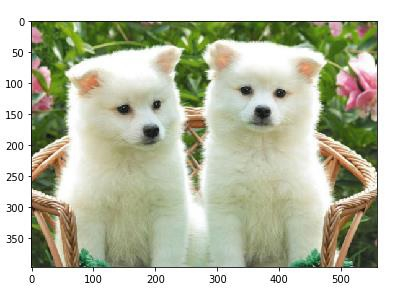# How to view the pixel values of an image using scikit-learn in Python?

Data pre-processing basically refers to the task of gathering all the data (which is collected from various resources or a single resource) into a common format or into uniform datasets (depending on the type of data).

Since real-world data is never ideal, there is a possibility that the data would have missing cells, errors, outliers, discrepancies in columns, and much more.

Sometimes, images may not be correctly aligned, or may not be clear or may have a very large size. The goal of pre-processing is to remove these discrepancies and errors.

To get the pixels of an image, a built-in function named ‘flatten’ is used. After the image is read, the pixel values are stored in the form of a dataframe. The function ‘flatten’ is used to convert the three dimensions of an RGB image into a single dimension and get the value of pixels into a dataframe.

Instead of printing the entire dataframe, the dimensions of the dataframe are printed. Let us take the example of uploading an image and getting the pixels present in the image as a dataframe using scikit-learn library −

## Example

from skimage import io
import pandas as pd
path = "path to puppy.PNG"
io.imshow(img)
print("Image printed on console")
my_df = pd.DataFrame(img.flatten())
print("The image pixels dimensions are ")
print(my_df.shape)

## Output

Image being read
Image printed on console
The image pixels dimensions are
(886104, 1)## Explanation

• The required libraries are imported.

• The path where the image is stored is defined.

• The ‘imread’ function is used to visit the path and read the image.

• The ‘imshow’ function is used to display the image on the console.

• The function ‘flatten’ is used to convert the three dimensions of an RGB image into a single dimension and get the value of pixels into a dataframe.

• Instead of printing the dataframe which has too many rows, the dimensions of the dataframe are displayed.

• The dataframe can be viewed using ‘print(my_df)’.

• The output is a dataframe with image pixel values printed on the console.

Updated on: 10-Dec-2020

403 Views